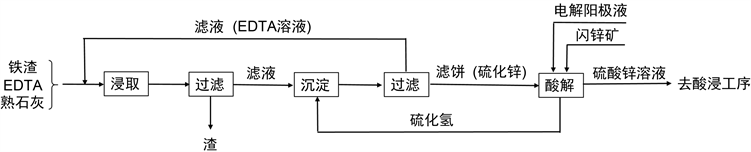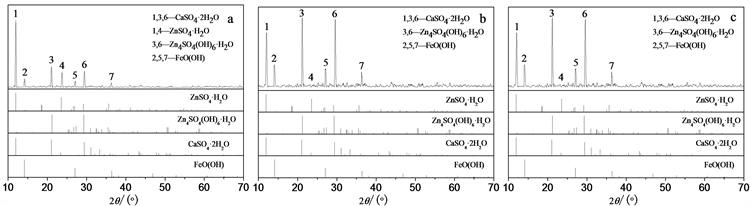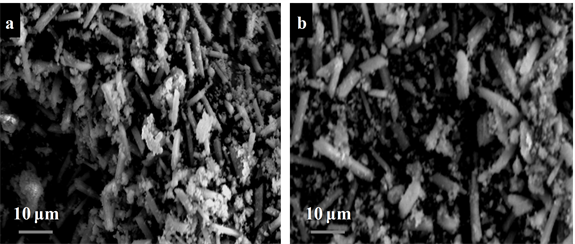#### 期刊菜单

EDTA浸取法从冶锌铁渣中回收锌
Retrieving Zinc from Iron-Zinc Slag by EDTA Leaching Method
DOI: 10.12677/AEP.2020.103041, PDF, HTML, XML, 下载: 533  浏览: 1,375  科研立项经费支持

Abstract: In order to investigate the leaching efficiency of zinc from iron-zinc slag via EDTA method, the ef-fects of initial concentration of ethylenediaminetetraacetic acid (EDTA), temperature , liquid-solid ratio and reaction time on the leaching rate and selectivity in process of leaching zinc from iron-zinc slag were studied. The results showed that EDTA method exhibited good leaching efficiency and selectivity of zinc, zinc was leached from iron-zinc slag preferentially. When the total EDTA concentration was 0.4 mol/L, the leaching temperature was 20˚C, the ratio of leaching agent volume to zinc dust mass was 6 L/kg and the leaching time was 2 h, the extraction rate of zinc was 99.77%.

1. 引言

2. 实验部分

2.1. 实验材料与仪器

2.2. 实验方法Figure 1. Technological process of recovering zinc from iron slag

2.2.1. EDTA浓度对浸取率及选择性的影响

2.2.2. 浸取液初始pH对浸出率及选择性的影响

2.2.3. 正交实验优化实验Table 1. Factors of the test for leaching zinc from iron-zinc slag by orthogonal experiment

2.2.4. EDTA与H2S的循环实验

3. 结果与讨论

3.1. EDTA浓度对浸取率及选择性的影响注：a：原铁渣；b：水浸铁渣；c：EDTA浸取铁渣

Figure 2. XRD patterns of iron-zinc slag leached by different methodsTable 2. Effect of EDTA concentration on the extraction amount of zinc, iron and calciumTable 3. Main chemical compositions of iron slag by pre/post-leachingFigure 3. The scanning electron micrographs of iron slag (a) and leaching residue (b)

3.2. 浸取液初始pH对浸出率及选择性的影响

${\text{H}}_{\left(4-n\right)}{\text{Y}}^{n-}+{\text{Zn}}^{2+}={\left[\text{ZnY}\right]}^{2-}+\left(4-n\right){\text{H}}^{+}$ (1)

$6{\text{H}}^{+}+{\text{Zn}}_{4}{\text{SO}}_{4}{\left(\text{OH}\right)}_{6}=4{\text{Zn}}^{2+}+{\text{SO}}_{4}^{2-}+6{\text{H}}_{2}\text{O}$ (2)

$3{\text{H}}^{+}+\text{FeO}\left(\text{OH}\right)={\text{Fe}}^{3+}+3{\text{H}}_{2}\text{O}$ (3)

${\text{H}}_{\left(4-n\right)}{\text{Y}}^{n-}+{\text{Fe}}^{3+}={\left[\text{FeY}\right]}^{-}+\left(4-n\right){\text{H}}^{+}$ (4)Table 4. Effect of pH on leaching rate and selectivity of zinc from iron slag

3.3. 正交实验优化Table 5. Effect of different leaching conditions on zinc extraction by EDTA method

3.4. EDTA与H2S的循环

${\left(\text{ZnY}\right)}^{2-}+{\text{H}}_{2}\text{S}=\text{ZnS}+{\text{H}}_{2}{\text{Y}}^{2-}$ (5)

$K=\left[{\text{H}}_{2}{\text{Y}}^{2-}\right]/\left[{\left(\text{ZnY}\right)}^{2-}\right]={K}_{{\text{H}}_{2}\text{S},1}{K}_{{\text{H}}_{2}\text{S},2}/\left({K}_{稳}{K}_{sp}{K}_{\text{EDTA},3}{K}_{\text{EDTA},4}\right)=2.588×{10}^{4}$

4. 结论

EDTA是很好的选择性浸取锌的浸取剂，浸取时要根据原料中水溶性锌和酸溶性锌的比例调节浸取液的初始pH值。通过正交实验优化显示EDTA的浓度和液固比对浸取影响高度显著，浸取时间对结果有一定的影响，浸取温度对结果影响较小，研究所得最佳浸取条件为EDTA浓度为0.4 mol/L、温度为20℃，固液比6 L/kg的条件下反应2 h，此时，锌的浸出率为99.77%。

NOTES

*通讯作者。

  魏昶, 李存兄. 锌提取冶金学[M]. 北京: 冶金工业出版社, 2013: 8.  邹晓勇, 彭清静, 吴贤文, 等. 锌产业技术及应用[M]. 北京: 化学工业出版社, 2019: 7.  Masambi, S., Dorfling, C. and Bradshaw, S. (2016) Comparing Iron Phosphate and Hematite Precipitation Processes for Iron Removal from Chloride Leach Solutions. Mineral Engi-neering, 98, 14-21. https://doi.org/10.1016/j.mineng.2016.07.001  王福生, 车欣. 浸锌渣综合利用现状及发展趋势[J]. 天津化工, 2010, 24(3): 1-3.  姚芝茂, 赵丽娜, 徐成. 锌治炼工业有价金属回收潜力与现状分析[J]. 中国有色冶金, 2011(1): 49-54.  闵小波, 张建强, 张纯, 等. 锌冶炼中浸渣锌还原浸出行为研究[J]. 有色金属科学与工程, 2015, 6(5): 1-6.  张亚平. 从浸锌渣还原铁粉中回收镓锗的工艺及机理[D]: [硕士学位论文]. 长沙: 中南大学, 2003.  王江伟, 彭清静. 氨浸法从冶锌铁渣中回收锌制备活性氧化锌[J]. 环境工程, 2019, 37(9): 165-170.  武汉大学编写组. 分析化学第二版[M]. 北京: 高等教育出版社, 1982: 10.  武汉大学编写组. 无机化学第二版[M]. 北京: 高等教育出版社, 1983: 4.  张锡瑜. 简明分析化学手册[M]. 北京: 北京大学出版社, 1981: 10.